• matlab图形处理matlab图形处理的功能 作了介绍，很详细
• matlab图形图像处理实现，人工智能，图像处理的文件
• 二维图形的基本函数 MATLAB 提供了非常丰富的图形绘制功能，并且每个图形元素（如坐标轴、曲线格式、文字等）都可以单独修改，而不影响图形的其它部分。
• Matlab图形图像处理函数详细列表-Matlab图形图像处理函数详细列表.rar Matlab图形图像处理函数详细列表.pdf
• matlab 图形处理教程 matlab 图形处理教程 matlab 图形处理教程
• Matlab处理图形图象的一些处理函数使用方法介绍
• 适合于学习matalb的编程者，可以直接使用并参考学习，主要是关于Matlab各种图形处理的程序，包含18个实用图形处理程序。
• MATLAB实现图形处理，很经典，需要的可以下下看啊
• matlab图形处理入门，讲的一些入门的知识，适合初学者看
• 基于matlab图形图像处理系统的实现基于matlab图形图像处理系统的实现
• MATLAB进行基础的图像处理 旋转剪切缩放等功能
• 图形窗口 MATLAB的所有图形都显示在特定的窗口中，称为图形窗口。可用figure创建一个新的图形窗口，每个图形窗口有一个编号，显示在图形窗口的左上角。如Figure 1等， 如果没创建图形窗口，则在调用画图命令时自动...
一基本绘图函数

平面曲线绘图
plot(Y)  如Y是实向量，则绘出横坐标值为序号，纵坐标值为Y的曲线，如Y是虚数，则横坐标值为Y的实部，纵坐标为Y的虚部的曲线，点与点之间用线段相连。如果Y是矩阵，则画出Y中各列相对于行号的曲线.

plot(X,Y), 如X，Y为同维实向量，则绘出横坐标为X纵坐标为Y的曲线
plot(X,Y, 'Linespec'), 按Linespec所规定的线型、标记点、颜色绘线。
其中Linespec为字符串，是下列字符的组合
线型： 实线 - ，长划虚线–，短虚线：，点划线-. ，
标记点：点.,  加号+，星号*，圈o，叉x，方块s，菱形d，上三角^, 下三角v,左三角<, 有三角>, 五角星p，六角星h
颜色：红r，绿g，蓝b，黄y，粉红m，青c，白w，黑k
甚至还可以设置线宽’LineWidth’,标记点边缘颜色’MarkerEdgeColor’，标记点填充颜色’MarkerFaceColor’，标记点大小’MarkerSize’ 如下例
plot(X,Y,'Linespec', '-bs', 'LineWidth',2,MarkerEdgeColor','y','MarkerFaceColor','y','MarkerSize',10)

如要画几条曲线在一张图上，只要在plot函数中依次输入即可，例
ploy(x1,y1,'r',x2,y2,'b')

如果几条曲线的横坐标值都相等为x，可以把几条曲线的纵坐标值组成一个矩阵Y的列向量，然后plot(x,Y)即可，这时各条曲线的颜色按照规定的次序循环表示。
如果几条曲线的纵坐标值都相等为y，可以把几条曲线的横坐标值组成一个矩阵X的列向量，然后plot(X,y)即可，这时各条曲线的颜色按照规定的次序循环表示。
不然，把各曲线的横坐标值组成一个矩阵X的列，列坐标组成矩阵Y的列，然后plot(X,Y).
要在图的正上方给出标题，可用title(‘标题’)实现，如
title('正弦函数图形','FontName','隶书','FontSize',20), 数学符号可用TeX语言输入，常用的几个：\leq 小于等于，\pi 圆周率，\int 积分号，\sum 求和号 \leftarrow 左箭头 \rightarrow右箭头
要加注坐标轴：
xlabel('横坐标的注')
ylabel('列坐标的注')

加注图例：
legend('字符串1','字符串2','字符串3',pos) 其中pos省却时图例位于右上角，0时为最佳位置，2为左上角，3为左下角，4为右下角，-1为图的右侧
如要使图例的边框不显示，可用legend('boxoff'), 如要图例不显示，可用legend('hide')
要在图形上的(x,y)处加写文本可用text(x,y,'加写的文本')
使用鼠标在指定位置写上文本：
gtext('文本')

这时在图形窗口出现一个随鼠标移动的十字交叉线，将鼠标指针移动到要写入的位置时，点击鼠标左键即可。
图形窗口
MATLAB的所有图形都显示在特定的窗口中，称为图形窗口。可用figure创建一个新的图形窗口，每个图形窗口有一个编号，显示在图形窗口的左上角。如Figure 1等， 如果没创建图形窗口，则在调用画图命令时自动创建一个图形窗口；如已有一个图形窗口，在缺省的情况下直接利用该窗口绘图，原窗口中如有图像则被覆盖，（如在hold on情况下则是图像叠加）；如存在多个打开的图形窗口，则将最后使用过得图形窗口作为当前窗口。可以用figure(n)表示将第n个图形窗口作为当前窗口（或者用鼠标点击需要指定的图形窗口）
用clf命令清除当前图形窗口中的内容，用shg命令将当前窗口放在最前面。
自定义坐标轴的显式刻度，例
t=-pi:pi/20:pi;
y=sin(t);
plot(t,y)
set(gca,'xtick',[-pi:pi/2:pi],'xticklabel',{'-pi','-pi/2','0','pi/2','pi'})
yong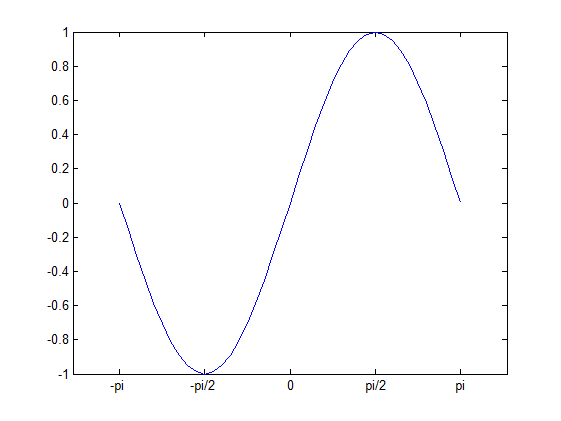用鼠标确定曲线上点的x坐标，在曲线上该点画红色星号，
t=0:pi/20:2*pi;
plot(t,cos(t));
hold on
set(gcf,'WindowButtonDownFcn',['p=get(gca,''CurrentPoint'');','px=p(1,1);py=cos(px);','plot(t,cos(t));','plot(px,py,''*r'');'])
axis square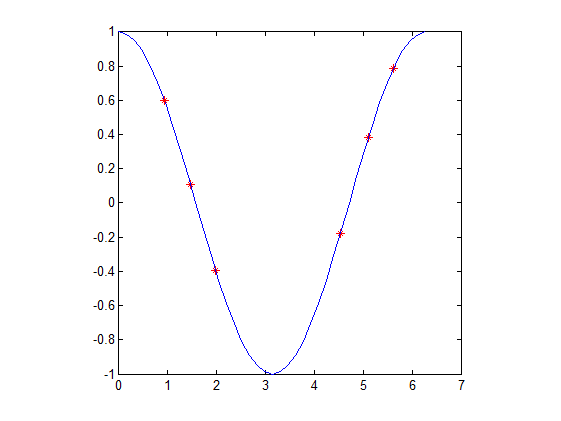要在一个图形窗口上画几个图形，可用subplot(m,n,p)把图形窗口分成m乘n个小窗口，并把第p个作为当前窗口，窗口的顺序是从左上角开始按行排列，然后用绘图函数，如plot等把图画在这个窗口中。
坐标轴的控制
用axis命令来控制坐标轴
axis([xmin,xmax,ymin,ymax]) 指定x与y轴的范围
axis tight 以数据的大小为坐标轴的范围
axis ij 使纵坐标向下为正
axis equal 使各坐标轴刻度增量相同
axis square 使坐标轴长度相同（刻度增量不必相同）
axis off 不显示坐标轴
其他平面绘图函数
对数坐标绘图：semilogx；semilogy； loglog
极坐标绘图： polar
双Y 轴图：plotyy；
条形：bar；例
x = -2.9:0.2:2.9;
bar(x,exp(-x.*x),'b')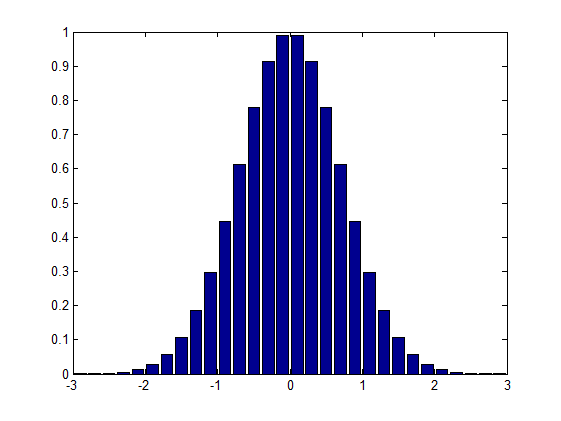符号数学的简易绘图函数easyplot，
ezpolar,ezplot3,ezcontour,ezmesh,ezsurf.ezsurfc；fplot例
syms x
ezplot(erf(x))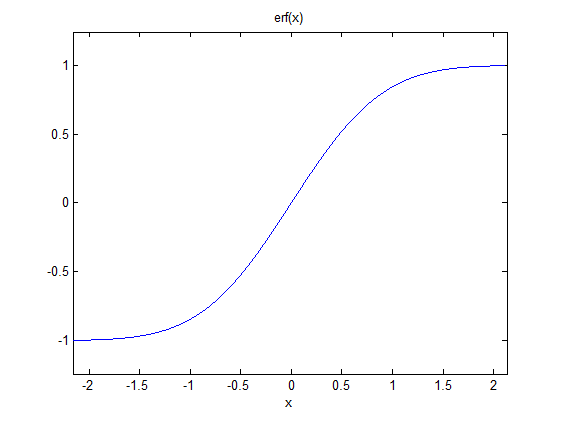syms t
ezpolar(1+cos(t))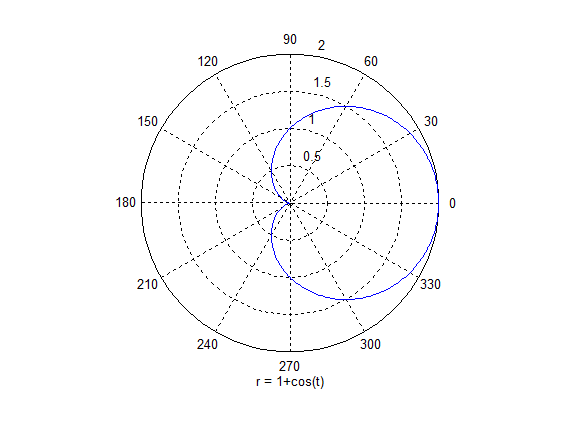syms t
ezplot3(sin(t),cos(t),t,[0,6*pi])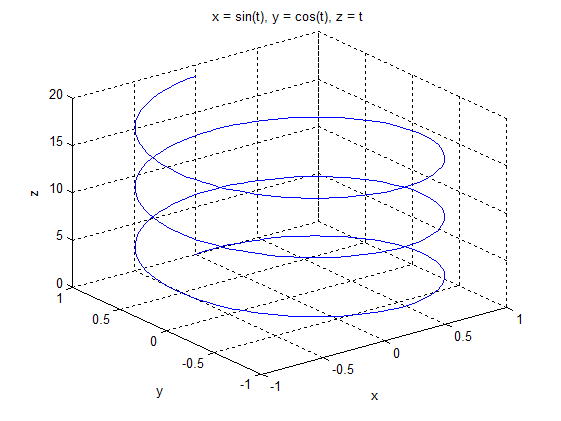syms t s
x=cos(s)*cos(t);
y=cos(s)*sin(t);
z=sin(s);
ezsurf(x,y,z,[0,pi/2,0,3*pi/2])
view(17,40)
shading interp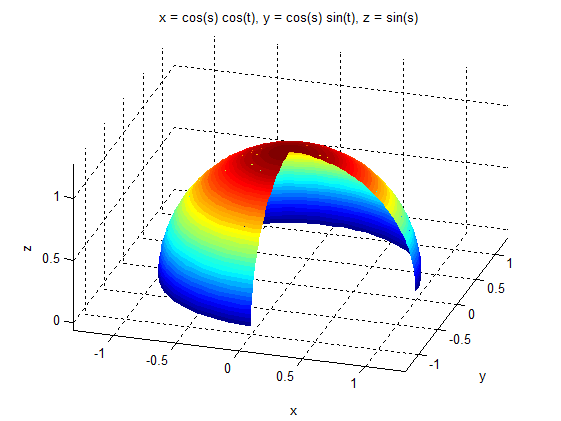fplot；可以画函数y=f(x)的图像
如定义一个函数（函数向量）


function Y = myfun(x)
Y(:,1) = 200*sin(x(:))./x(:);
Y(:,2) = x(:).^2;

再建立一个函数句柄
fh = @myfun;

则可画图像
fplot(fh,[-20 20])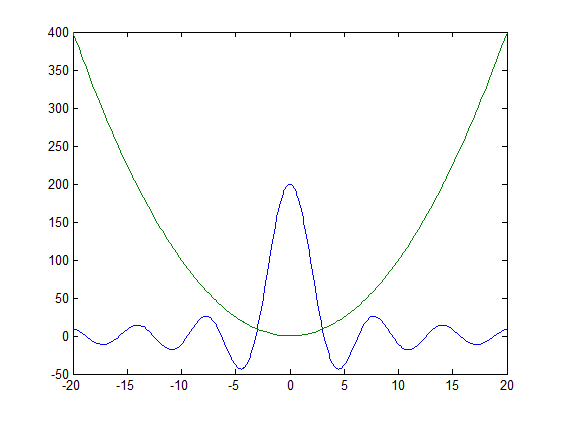三维条形图： bar3；
水平条形图： barh； 三维水平条形图：bar3h；
n组条形图 group
饼图：pie ； 三维饼图pie3；
直方图： hist；玫瑰图，在极坐标下的直方图: rose; 例
theta = 2*pi*rand(1,50);
rose(theta)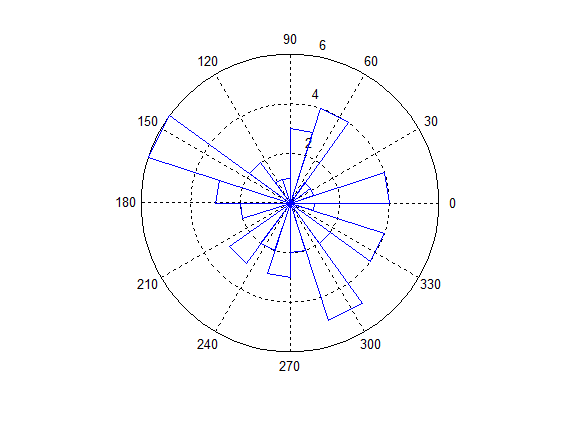杆状图： stem；三维杆状图 stem3
阶梯图：stairs；
彩色分散点图：scatter；可以改变点的大小和颜色的分散点图；
填充的2-D图函数area；例
Y = [1, 5, 3;
3, 2, 7;
1, 5, 3;
2, 6, 1];
area(Y)
grid on
colormap summer
set(gca,'Layer','top')
title 'Stacked Area Plot'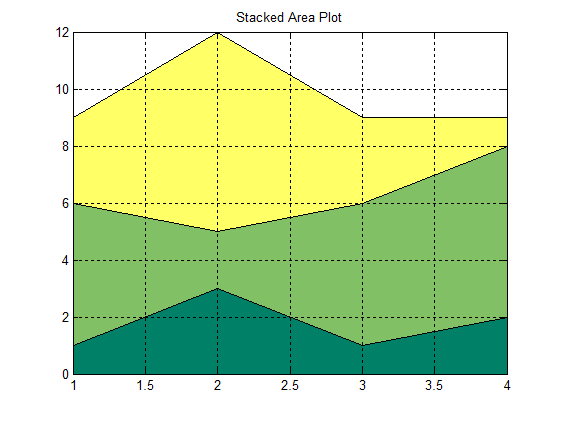方向及向量图
罗盘图compass；
羽毛图feather；
箭图quiver；
3维箭图quiver3；
三维空间的绘图函数
画空间曲线：plot3
画空间曲面函数
生成网格矩阵：
meshgrid；
mesh;surf;
meshc;surfc; 带有等位线(contour)的图
meshz; 带有帘(curtain)的图
pcolor 用颜色代表高度的平面图
surfl; 可指定光照效果的图
例
[x,y] = meshgrid(-3:1/8:3);
z = peaks(x,y);
surfl(x,y,z);
shading interp
colormap(gray);
axis([-3  3  -3  3  -8  8])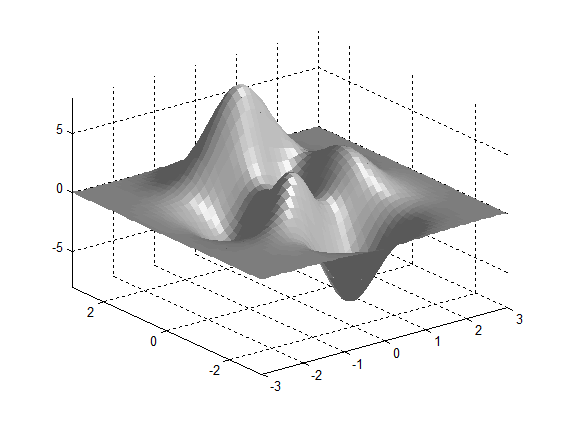surface; 低级绘图函数
画多面体填充颜色的函数
fill, 画多边形填充图
fill3, 画多面体填充表面图
例
t = (1/16:1/8:1)'*2*pi;
x = sin(t);
y = cos(t);
fill(x,y,'r')
axis square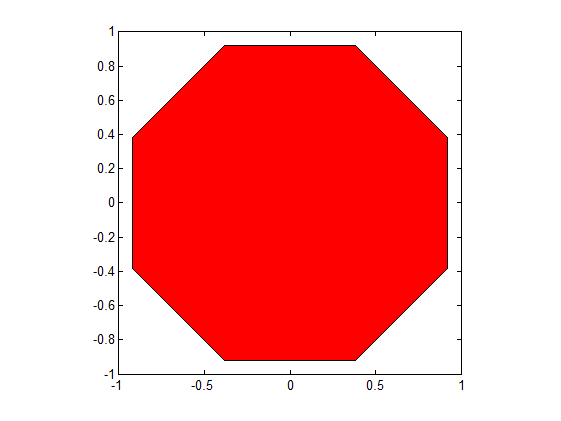画旋转曲面：
cylinder
t = 0:pi/10:2*pi;
cylinder(2+cos(t),30);  %在圆周上取30个网格点
grid off %去掉坐标网格线
axis off %去掉坐标轴
colormap('default') %采用默认色彩图jet
shading interp %使图形表面为透明，无网格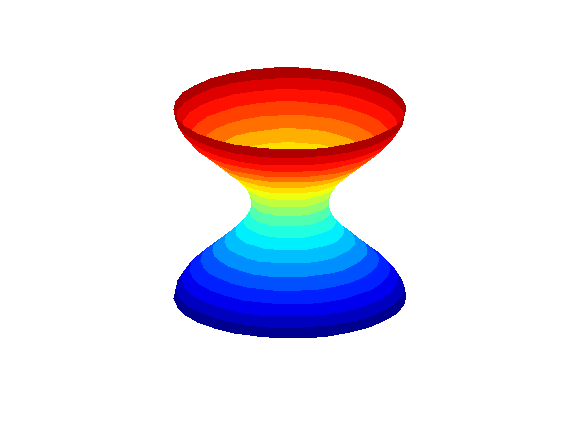色彩图共有以下几种
hsv        - Hue-saturation-value color map.
hot        - Black-red-yellow-white color map.
gray       - Linear gray-scale color map.
bone       - Gray-scale with tinge of blue color map.
copper     - Linear copper-tone color map.
pink       - Pastel shades of pink color map.
white      - All white color map.
flag       - Alternating red, white, blue, and black color map.
lines      - Color map with the line colors.
colorcube  - Enhanced color-cube color map.
vga        - Windows colormap for 16 colors.
jet        - Variant of HSV.
prism      - Prism color map.
cool       - Shades of cyan and magenta color map.
autumn     - Shades of red and yellow color map.
spring     - Shades of magenta and yellow color map.
winter     - Shades of blue and green color map.
summer     - Shades of green and yellow color map.

也可以自定义色彩图
画椭球面
ellipsoid(x0,y0,z0, a,b,c) %画中心在(x0,y0,z0), 三个半长轴分别为a，b，c的椭球面

动画制作之例：
for k = 1:16 % 用for循环制作图像
plot(fft(eye(k+16)))
axis equal
M(k) = getframe;
end

movie(M,30)  %放映动画30次



展开全文数学建模...

# matlab处理图形matlab 订阅